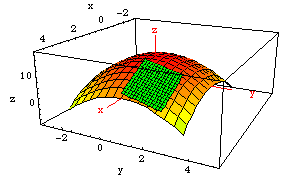# Plotting Tangent Planes to a Surface in MathematicaCopyright © 2001 by James F. Hurley, University of Connecticut, Department of Mathematics, Unit 3009, Storrs CT 06269-3009.  All rights reserved.

Consider the function f(x, y) = x/y. Mathematica's built-in command Plot3D can plot the graph if you avoid the xz-plane on which f is discontinuous. As usual, generate the plot by placing the cursor at the end of the code and hitting the Enter key.

$\text{}{\text{(*}}{\text{}}{\mathrm{Mathematica}}{\text{}}{\mathrm{Routine}}{\text{}}{\mathrm{to}}{\text{}}{\mathrm{plot}}{\text{}}{\mathrm{graph}}{\text{}}{\mathrm{of}}{\text{}}{a}{\text{}}\text{}\text{}{\mathrm{surface}}{\text{}}{z}{\text{}}{=}{\text{}}f\left(x,\text{}y\right){*}{\right)}{\text{}}{\text{}}{\text{}}{\text{}}F\left[\text{x_},\text{}\text{y_}\right]\text{}:=\text{}x/y;\text{}\text{}\mathrm{Plot3D}\left[\text{}F\left[x,\text{}y\right],\text{}\left\{x,\text{}1/4,\text{}3\right\},\text{}\left\{y,\text{}1/4,\text{}3\right\},\text{}\text{}\mathrm{AxesLabel}\text{}->\text{}\left\{"x",\text{}"y",\text{}"z"\right\}\text{}\right]\text{}$

To plot the tangent plane to this surface at a point such as P(2, 1, 2), the first step is to calculate the partial derivatives f /∂x and f /y at P. That is easy for this function:

$\frac{\partial f}{\partial x}$ = $\frac{1}{y}$  = 1 at (2, 1, 2)  and   $\frac{\partial f\text{}}{\partial y}$= - $\frac{x}{{y}^{2}}$ = -  2 at (2, 1, 2).

So the equation of the tangent plane to the graph of
f at P is z - 2 = (x - 2) - 2(y - 1).  The next routine plots that plane in yellow, and then redraws it with the Cartesian coordinate axes.

$\text{}{\text{(*}}{\text{}}{\mathrm{Mathematica}}{\text{}}{\mathrm{Routine}}{\text{}}{\mathrm{to}}{\text{}}{\mathrm{plot}}{\text{}}{\mathrm{graph}}{\text{}}{\mathrm{of}}{\text{}}{a}{\text{}}{\mathrm{surface}}{\text{}}{\text{}}{\text{}}{z}{\text{}}{=}{\text{}}f\left(x,\text{}y\right){,}{\text{}}\mathrm{with}\mathrm{coordinate}\mathrm{axes}\mathrm{and}\mathrm{tangent}\mathrm{plane}{\text{*)}}{\text{}}{\text{}}\text{}\text{}\text{}a\text{}:=\text{}-1;\text{}\text{}b\text{}:=\text{}2;\text{}\text{}c\text{}:=\text{}-1;\text{}\text{}\text{}d\text{}:=\text{}2;\text{}\text{}e\text{}:=\text{}-1;\text{}\text{}f\text{}:=\text{}5;\text{}\text{}\text{}T\left[\text{x_},\text{}\text{y_}\right]\text{}:=\text{}2\text{}+\text{}\left(x\text{}-\text{}2\right)\text{}-\text{}2*\left(y\text{}-\text{}1\right)\text{}{\text{}}{\mathrm{planeplot}}{\text{}}{=}{\text{}}{\mathrm{Graphics3D}}{\left[}{\text{}}{\text{}}{\mathrm{Plot3D}}{\left[}{\text{}}\left\{\text{}T\left[x,\text{}y\right],\text{}\mathrm{RGBColor}\left[1,\text{}1,\text{}0\right]\right\},\text{}\text{}\text{}\left\{x,\text{}1,\text{}3\right\},\text{}\left\{y,\text{}0,\text{}2\right\},\text{}\text{}\text{}\mathrm{AxesLabel}\text{}->\text{}\left\{"x",\text{}"y",\text{}"z"\right\}{\text{}}\text{}{\right]}{\text{}}{\text{}}{\text{}}{\right]}\text{}{;}\text{}\text{}\mathrm{coordaxes}\text{}=\text{}\mathrm{Graphics3D}\left[\text{}\left\{\text{}\text{}\left\{\text{}\mathrm{RGBColor}\left[1,\text{}0,\text{}1\right],\text{}\text{}\text{}\mathrm{Line}\left[\left\{\left\{a,\text{}0,\text{}0\right\},\text{}\left\{b,\text{}0,\text{}0\right\}\right\}\right],\text{}\text{}\mathrm{Text}\left["x",\text{}\left\{b\text{}+\text{}\text{.25},\text{}0,\text{}0\right\}\right]\text{}\right\},\text{}\text{}\left\{\text{}\mathrm{RGBColor}\left[1,\text{}0,\text{}1\right],\text{}\text{}\text{}\mathrm{Line}\left[\left\{\left\{0,\text{}c,\text{}0\right\},\text{}\left\{0,\text{}d,\text{}0\right\}\right\}\right],\text{}\text{}\mathrm{Text}\left["y",\text{}\left\{0,\text{}d\text{}+\text{}\text{.25},\text{}0\right\}\right]\text{}\right\},\text{}\text{}\left\{\text{}\mathrm{RGBColor}\left[1,\text{}0,\text{}1\right],\text{}\text{}\text{}\mathrm{Line}\left[\left\{\left\{0,\text{}0,\text{}e\right\},\text{}\left\{0,\text{}0,\text{}f\right\}\right\}\right],\text{}\text{}\mathrm{Text}\left["z",\text{}\left\{0,\text{}0,\text{}f\text{}+\text{}1\right\}\right]\text{}\right\}\text{}\text{}\right\}\text{}\right];\text{}{\text{}}\mathrm{Show}\left[\mathrm{planeplot},\text{}\mathrm{coordaxes},\text{}\mathrm{ViewPoint}\text{}->\text{}\left\{2,\text{}1,\text{}1\right\}\right]{\text{}}{\text{}}{\text{}}\text{}$

Plotting a surface and its tangent plane at a point. To combine two earlier Mathematica plots, ask Mathematica to Show them to you. The following command does so, and also asks Mathematica to redraws the first plot. Notice that even with an increased number of points plotted, the abruptness of the surface makes the tangent plane appear to cut through the surface.

surfplot = Graphics3D[
Plot3D[ {F[x, y], Hue[(x^2 + y^2)/30]},
{x, 1/4, 3}, {y, 1/4, 3},
PlotPoints -> 50,
AxesLabel -> {"x", "y", "z"} ] ];
Show[surfplot, planeplot, coordaxes, ViewPoint -> {2, .5, 1}, Lighting -> False]

A tamer example. Consider the function g with formula g(x, y) = 9 - ${x}^{2}$ - ${y}^{2}$. The graph of g is a paraboloid (of revolution about the z-axis), for which the following Mathematica routine generates a good picture.

$g\left[\text{x_},\text{}\text{y_}\right]\text{}:=\text{}9\text{}-\text{}x^2\text{}-\text{}y^2;$

$\text{}\mathrm{Plot3D}\left[\text{}g\left[x,\text{}y\right],\text{}\left\{x,\text{}-3,\text{}3\right\},\text{}\left\{y,\text{}-3,\text{}3\right\},\text{}\text{}\text{}\mathrm{AxesLabel}\text{}->\text{}\left\{"x",\text{}"y",\text{}"z"\right\}\text{}\right]\text{}$

Let's generate a plot of the tangent plane to this surface at the point (2, 1, 4). The partial derivatives there are

$\frac{\partial f}{\partial x}$ = - 2x = - 4     and      $\frac{\partial x}{\partial y}$ = - 2y = - 2.

The equation of the tangent plane at (2, 1, 4) is therefore

z - 4 = - 4(x - 2) - 2(y - 1).

The following routine plots that plane, and then replots it with the addition of red coordinate axes. (The initial settings of the parameters
a, b, ..., f reflect some experimentation with the figure the results from executing the routine.)

$a\text{}:=\text{}-3;$

$\text{}b\text{}:=\text{}4;$

$\text{}c\text{}:=\text{}-3;\text{}$

$\text{}d\text{}:=\text{}4;$

$\text{}e\text{}:=\text{}-9;$

$\text{}f\text{}:=\text{}12;$

$\text{}T\left[\text{x_},\text{}\text{y_}\right]\text{}:=\text{}4\text{}-\text{}4\left(x\text{}-\text{}2\right)\text{}-\text{}2\left(y\text{}-\text{}1\right)$

$\text{}\mathrm{planeplot}\text{}=\text{}\text{}\text{}\mathrm{Graphics3D}\left[\text{}\text{}\mathrm{Plot3D}\left[\text{}\left\{T\left[x,\text{}y\right],\mathrm{RGBColor}\left[0,\text{}1,\text{}0\right]\right\},\text{}\text{}\text{}\left\{x,\text{}1,\text{}3\right\},\text{}\left\{y,\text{}0,\text{}2\right\},\text{}\text{}\text{}\mathrm{AxesLabel}\text{}->\text{}\left\{"x",\text{}"y",\text{}"z"\right\}\text{}\right]\text{}\text{}\text{}\right];$

$\text{}\mathrm{coordaxes}\text{}=\text{}\mathrm{Graphics3D}\left[\left\{\text{}\text{}\left\{\mathrm{RGBColor}\left[1,\text{}0,\text{}0\right],\text{}\text{}\text{}\mathrm{Line}\left[\left\{\left\{a,\text{}0,\text{}0\right\},\text{}\text{}\text{}\left\{b,\text{}0,\text{}0\right\}\right\}\right],\text{}\text{}\mathrm{Text}\left["x",\text{}\left\{1.1\text{}b,\text{}0,\text{}0\right\}\right]\right\},\text{}\text{}\left\{\mathrm{RGBColor}\left[1,\text{}0,\text{}0\right],\text{}\text{}\text{}\mathrm{Line}\left[\left\{\left\{0,\text{}c,\text{}0\right\},\text{}\text{}\text{}\left\{0,\text{}d,\text{}0\right\}\right\}\right],\text{}\text{}\mathrm{Text}\left["y",\text{}\left\{0,\text{}d\text{}+\text{}1,\text{}0\right\}\right]\right\},\text{}\text{}\left\{\mathrm{RGBColor}\left[1,\text{}0,\text{}0\right],\text{}\text{}\text{}\mathrm{Line}\left[\left\{\left\{0,0,e\right\},\text{}\text{}\left\{0,\text{}0,\text{}2.5\text{}f\right\}\right\}\right],\text{}\text{}\mathrm{Text}\left["z",\text{}\left\{0,\text{}0,\text{}1.6\text{}f\right\}\right]\right\}\text{}\text{}\right\}\right];$

$\text{}\mathrm{Show}\left[\mathrm{planeplot},\text{}\mathrm{coordaxes}\right]\text{}$

The following command draws the preceding two separate plots together (after redrawing the first one). Try it!

$\mathrm{surfplot}\text{}=\text{}\mathrm{Graphics3D}\left[\text{}\text{}\mathrm{Plot3D}\left[\text{}\left\{g\left[x,\text{}y\right],\text{}\mathrm{Hue}\left[\left(x^2\text{}+\text{}y^2\right)/80\right]\right\},\text{}\text{}\text{}\left\{x,\text{}-3,\text{}3\right\},\text{}\left\{y,\text{}-3,\text{}3\right\},\text{}\text{}\text{}\mathrm{AxesLabel}\text{}->\text{}\left\{"x",\text{}"y",\text{}"z"\right\}\text{}\right]\text{}\right]\text{}$

$\mathrm{Show}\left[\mathrm{surfplot},\text{}\mathrm{planeplot},\text{}\mathrm{coordaxes},\text{}\mathrm{Lighting}\text{}->\text{}\mathrm{False},\text{}\mathrm{ViewPoint}\text{}->\text{}\left\{2,\text{}1,\text{}\text{.75}\right\}\right]$

$⁃\mathrm{Graphics3D}⁃$$⁃\mathrm{Graphics3D}⁃$

Converted by Mathematica  (June 10, 2003)